H o w 105 116 032 119 097 115 032 109 111 114 101 032 102 117 110 032 105 110 032 104 101 108 108 013010 m a n y ofus have set upon t h e for searching road same g o l d e n success regardless of the weight o f t h e withgrinning and eyed bright challenge s w a g s come strong g t b e e u e p t r s eventuallypace weakens first a s w e rare extraordinarily not are we that realize w e the same wornpaths of those t b a r e r e f e a o dfamily your r n n t g e o i a o t g u a u g l s u p h s no better than them n e tplans p i o h o q p y a s u l o d s e e u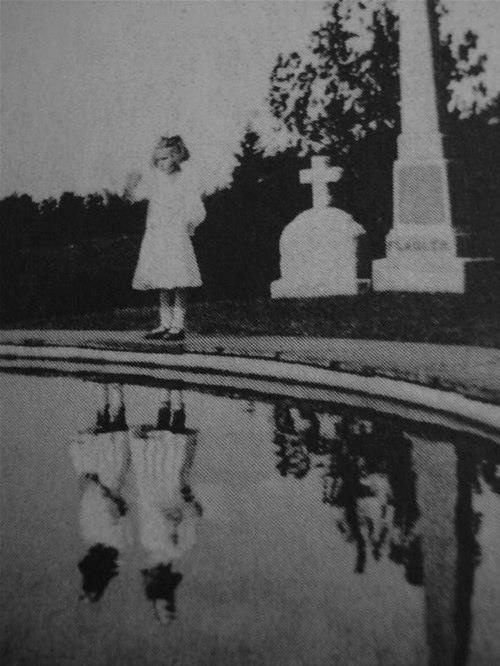remember you i we are only how d b t o l o n y plan t b or right you w u l o t e w y r where f a o s a t s u e r it and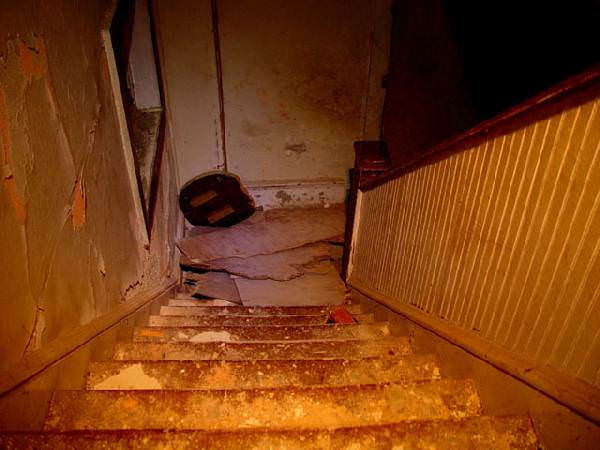behind look as increep to starts doubt e t h e y n o w and weren't you supposed to be anadult now where are they now i l y n e o f u c t l a o o r s r e i n ra g i l g o a h n r t e e is it even worth and thewalls t h et r o u b l it's butescape from e f y is this maybe i o g n u o e o the world has k d e e e e n p n o bridges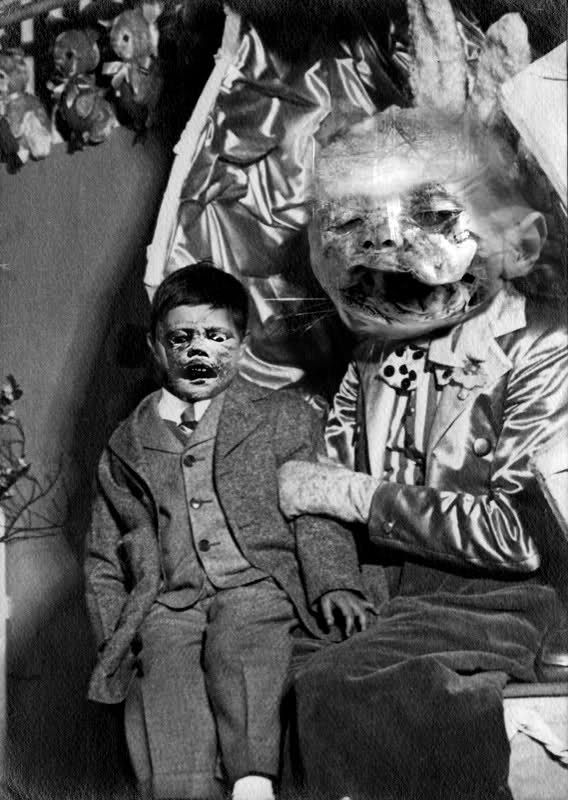burnt o u w u g under debt h g in w h b a i h a u n lyou s l d r d e d e l o i loan i l o c e d f w t d p ocar expensive yourwife beatufiul yourjob successful your y i y r n o t o s o u y u not excuses they are truths w r a only your i h c a n a c s a f b r s d r hsettling r u e a e o y d t s w p l i o realizations u t l o a t c u tell that r i s w h e e c n i y e but where is your glowing h g t e s u i r e m o o s perfect up end didnt it if what so n n there are enough of the prosperous what we need is some n the for overqualified even maybe you dont bill the f d y i o o t w u you n r a t n r k d o n good we have it d e h d e o e s w n us of rest the show to failures some through a challange you only young and stupid the naivete ofyouth t o w h f h o gravity the understand to e u c r g a e h m t e a of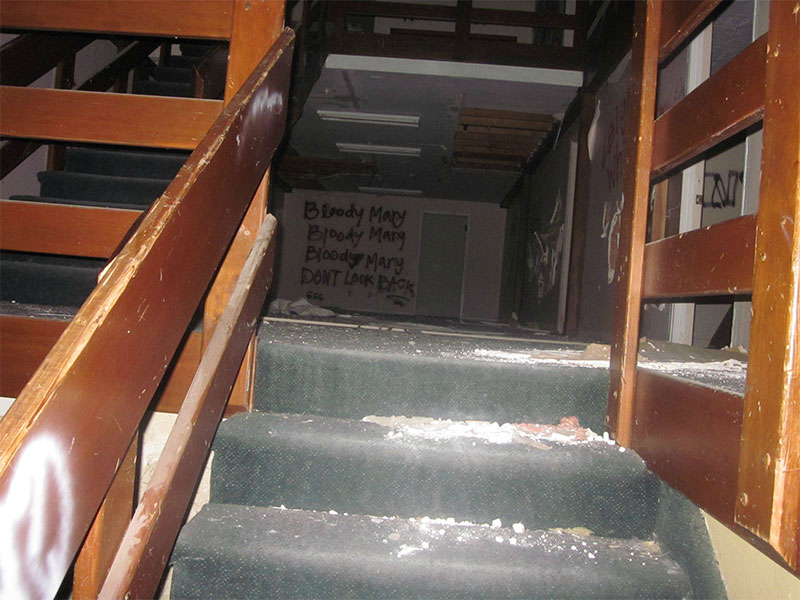comfort the that beginning t you were prepared for r f h b y e a e u o i that luck takes you back to t u y l h then o u o u r p e i n n o i g w s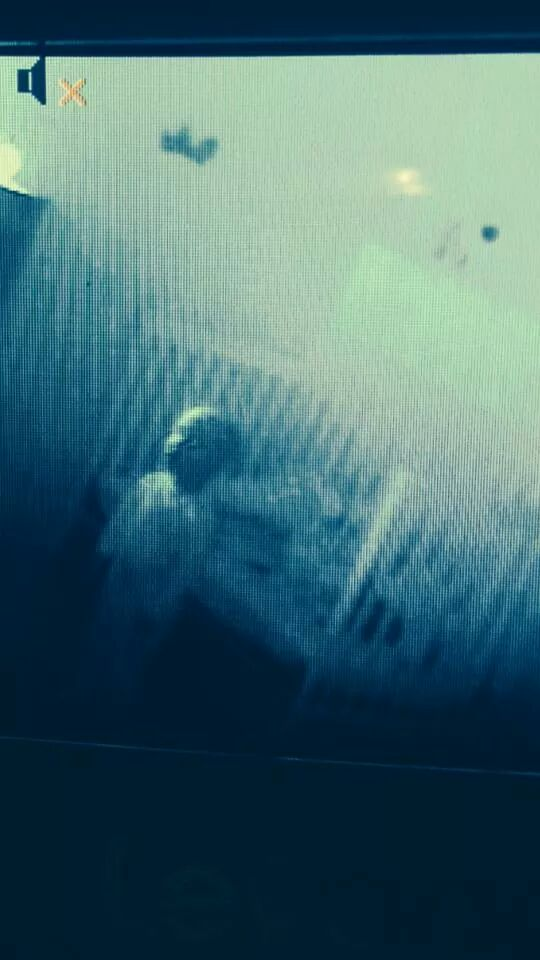child motherless a like crying sniveling nails with ground rawclawing b e t risks of hardshippity you but no one t t a a b e h n l a r e d l special t than we a n s n o d t a n t a h r e i e y s e.you for much too always wasy for the weak o w u estruggles own our have